#### Dijkstra's algorithm

Up to this point, there has been no reason to prefer one action over any other in the search. Section 2.3 will formalize optimal discrete planning and will present several algorithms that find optimal plans. Before going into that, we present a systematic search algorithm that finds optimal plans because it is also useful for finding feasible plans. The result is the well-known Dijkstra's algorithm for finding single-source shortest paths in a graph , which is a special form of dynamic programming. More general dynamic programming computations appear in Section 2.3 and throughout the book.

Suppose that every edge,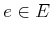, in the graph representation of a discrete planning problem has an associated nonnegative cost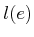, which is the cost to apply the action. The costcould be written using the state-space representation as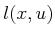, indicating that it coststo apply actionfrom state. The total cost of a plan is just the sum of the edge costs over the path from the initial state to a goal state.

The priority queue,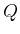, will be sorted according to a function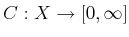, called the cost-to-come. For each state, the value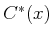is called the optimal2.1 cost-to-come from the initial state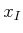. This optimal cost is obtained by summing edge costs,, over all possible paths fromtoand using the path that produces the least cumulative cost. If the cost is not known to be optimal, then it is written as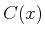.

The cost-to-come is computed incrementally during the execution of the search algorithm in Figure 2.4. Initially,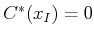. Each time the state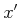is generated, a cost is computed as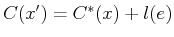, in which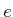is the edge fromto(equivalently, we may write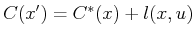). Here,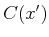represents the best cost-to-come that is known so far, but we do not write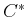because it is not yet known whetherwas reached optimally. Due to this, some work is required in line 12. Ifalready exists in, then it is possible that the newly discovered path tois more efficient. If so, then the cost-to-come valuemust be lowered for, andmust be reordered accordingly.

When doesfinally becomefor some state? Onceis removed fromusing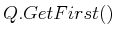, the state becomes dead, and it is known thatcannot be reached with a lower cost. This can be argued by induction. For the initial state,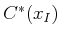is known, and this serves as the base case. Now assume that every dead state has its optimal cost-to-come correctly determined. This means that their cost-to-come values can no longer change. For the first element,, of, the value must be optimal because any path that has a lower total cost would have to travel through another state in, but these states already have higher costs. All paths that pass only through dead states were already considered in producing. Once all edges leavingare explored, thencan be declared as dead, and the induction continues. This is not enough detail to constitute a proof of optimality; more arguments appear in Section 2.3.3 and in . The running time is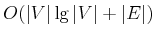, in which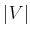and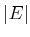are the numbers of edges and vertices, respectively, in the graph representation of the discrete planning problem. This assumes that the priority queue is implemented with a Fibonacci heap, and that all other operations, such as determining whether a state has been visited, are performed in constant time. If other data structures are used to implement the priority queue, then higher running times may be obtained.

Steven M LaValle 2020-08-14